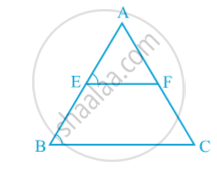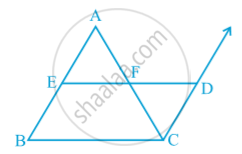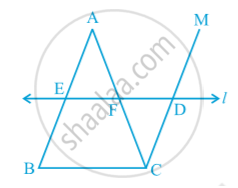SSC (English Medium) Class 9Maharashtra State Board
Share

# Concept: The Mid-point Theorem

#### text

Draw a triangle and mark the mid-points E and F of two sides of the triangle. Join the points E and F in following fig.Measure EF and BC. Measure ∠ AEF and ∠ ABC. EF = 1/2 BC and ∠ AEF = ∠ ABC
so, EF || BC

#### theorem

Theorem : The line segment joining the mid-points of two sides of a triangle is parallel to the third side.
Observe following Fig.  in which E and F are mid-points of AB and AC respectively and CD || BA.∆ AEF ≅ ∆ CDF (ASA Rule)
So, EF = DF and BE = AE = DC
Therefore, BCDE is a parallelogram.
This gives EF || BC.
In this case, also note that EF = 1/2 ED = 1/2 BC.

#### theorem

Theorem : The line drawn through the mid-point of one side of a triangle, parallel to another side bisects the third side.
In following fig.Observe that E is the mid-point of AB, line l is passsing through E and is parallel to BC and CM || BA.
Prove that AF = CF by using the congruence of ∆ AEF and ∆ CDF.

#### description

• Theorem of midpoints of two sides of a triangle
• Converse of midpoint theroem

### Shaalaa.com

Mid-point theorem [00:15:12]
S
0%

S